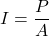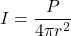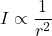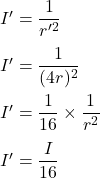## How does the intensity of a sound wave change if the distance from the source is increased by a factor of 4? A. The intensity de

Question

How does the intensity of a sound wave change if the distance from the
source is increased by a factor of 4?
A. The intensity decreases by a factor of 4.
B. The intensity increases to by a factor of 16.
c. The intensity decreases by a factor of 16.
D. The intensity increases by a factor of 4.

in progress 0
4 months 2021-09-05T05:51:47+00:00 1 Answers 3 views 0

The new intensity decreases by a factor of 16.

Explanation:

The intensity of sound wave is given by :P is power

A is areaor, r is distance from the source

If the distance from the  source is increased by a factor of 4, r’ = 4r

So,So, the new intensity decreases by a factor of 16.